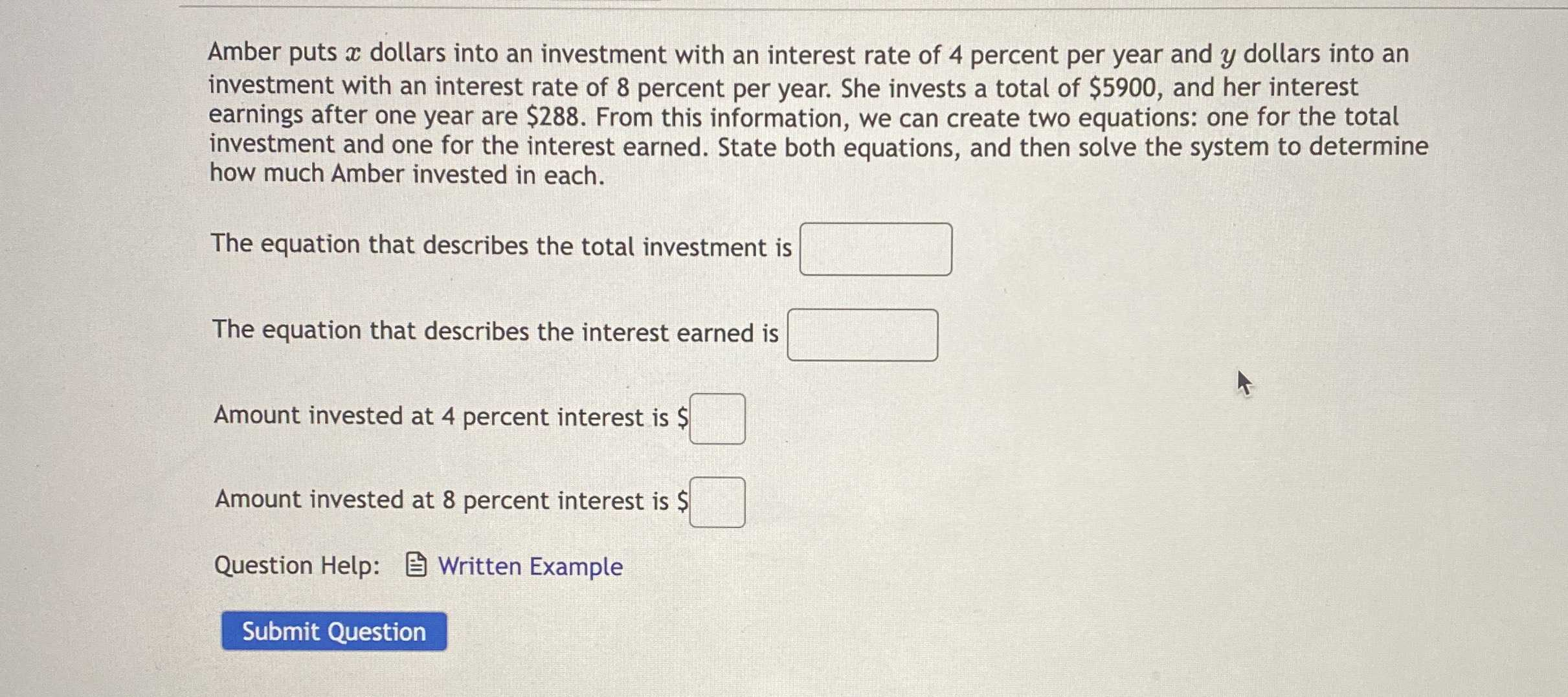### Still have math questions?

Algebra
QuestionAmber puts $$x$$ dollars into an investment with an interest rate of $$4$$ percent per year and $$y$$ dollars into an investment with an interest rate of $$8$$ percent per year. She invests a total of $$\ 5900$$ , and her interest earnings after one year are $$\ 288$$ . From this information, we can create two equations: one for the total investment and one for the interest earned. State both equations, and then solve the system to determine how much Amber invested in each. The equation that describes the total investment is The equation that describes the interest earned is Amount invested at $$4$$ percent interest is Amount invested at $$8$$ percent interest is

total investment: $$x+ y= 5900$$
total interest: $$0.04x+ 0.08y= 288$$
$$x= 4600\\y= 1300$$